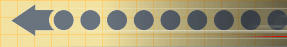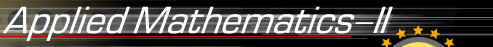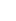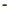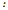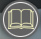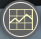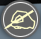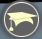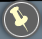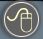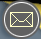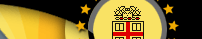Differential Equations on the web: Lamar University, Paul's Online Notes Differential Equations , by Khan Academy Eric Wisstein's World Of Mathematics WolframAlpha Widgets General Differential Equation Solver Package deSolve Solving IVPs in R Package onSolver Solving of differential equations online Maths Tools EqWorld The World Of Mathematical Equations S.O.S. Mathematics Differential Equations Differential Equations MIT Open Course Ware IDEA Internet Differential Equations Activities MDEP, by Jim Buchanan Recent development of various computers, from the personal computer to the super computer, involves rapid change in computer architecture and advancement in software engineering. There is a proliferation of solvers which focus on differential equations, ranging from simple numeric solvers to very comprehensive computer algebra software (CAS) solvers. These packages are very helpful in education, numerical simulation and applications of differential equations and more is being developed every day. This is not a complete and comprehensive listing, we pay attention to the most popular packages. Maxima is a descendant of DOE Macsyma, the oldest freely redistributable CAS Matlab®, by MathWorks with dfield8 by John C. Polking Solving ODEs in Matlab MIT Getting Started, by the Mathworks Solving ODEs in Matlab , by TAMU Matlab Tutorial, by Brown University Matlab Tutorial, by Michigan Tech Matlab Tutorial, by the University of Michigan Matlab Tutorial, by Mark S. Gockenbach ODE Software, by John C. Polking from Rice University MuPAD, by the MuPAD Research Group Octave, is freely redistributable software similar to Matlab Maple™, by Maplesoft with applications to ODE Maple Tutorial by Mark S. Gockenbach Maple Tutorial, by Maplesoft Solving ODEs with Maple , by Princeton University Solving PDEs with Maple , by Studocu Maple links , by Rensselaer Polytechnic Institute Maple Tutorial , by Whitman College Mathcad, by MathSoft Mathematica®, by Wolfram Reserch, Inc. Differential Equation using DSolve by John Boccio Mathics Free alternative to Mathematica Wolfram Alpha Understanding Differential Equations using Mathematica; CODEE Journal Differential Equations, by the University of California, Irvine Mathematica Tutorial, by Colgate University Advanced Numerical Differential Equations, by King Fahd University of Petroleum & Minerals R (free software) Octave (free software) Sage (free computer algebra system) SymPy (free computer algebra system) Python (free software) matlab (and its free version, Octave) is used extensively in the scientific computing community, is fairly easy to learn, and quite robust offering packages for visualization, numerical linear algebra, numerical differential equations and limited symbolic computation. MuPad is a computer algebra system within matlab based on Maple. The matlab Live Editor provides a new way to create, edit, and run matlab code. It creates, edits, and run code in a single interactive environment. Other small packages: MathView by Waterloo Maple Inc. DEPAC, by L. F. Shampine and H.A. Watts SDRIV, contains three packages ODEPACK Ordinary Differential Equation Solvers MVT, (the Mathematical Visualization Toolkit) by the University of Colorado Winplot, by Richard Parris, University of Hawaii ODE Toolkit, nice Java application for ODEs GeoGebra is the free and multi-platform, open-source dynamic mathematics software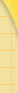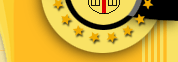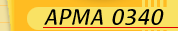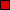ReadingSyllabusGradingExams & SolutionsHomeworkUtilities/softwareapplicationsContactComputingMain WebsiteAPMA0330APMA0340APMA1210APMA2140CS0520